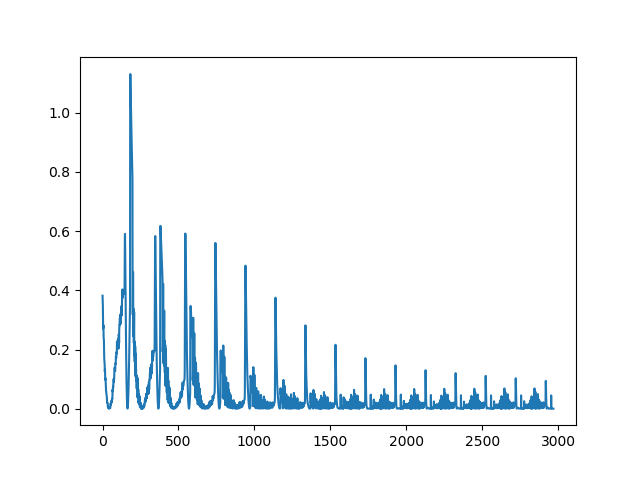# Loss function problem

Hi everyone, i have a problem about loss function. I have 2 tensors and x=[23177,1,3] and y=[23177,1]. They are my special datasets. So when I turn them into a dataloader:

``````train_dataset = TensorDataset(train_x, train_y)
``````

and my criterion and optimizer:

``````criterion = nn.MSELoss()
``````

and my nn class is:

``````class Modele(nn.Module):
def __init__(self, D_in, H1, H2, D_out):
super().__init__()
self.linear1 = nn.Linear(D_in, H1)
self.linear2 = nn.Linear(H1, H2)
self.linear3 = nn.Linear(H2, D_out)
def forward(self, x):
x = F.relu(self.linear1(x))
x = F.relu(self.linear2(x))
x = self.linear3(x)
return x

``````

and my computation loop:

epochs=15
for e in range(epochs):

``````    running_loss = 0.0
running_corrects = 0.0
val_running_loss = 0.0
val_running_corrects = 0.0

for inputs,out in train_generator:

loss = criterion(model(inputs), out)
#_,preds=torch.max(outputs,1)
#outputss.append(preds.max().detach().numpy())
losses.append(loss)
loss.backward()
optimizer.step()

#outputss.append(outputs.detach().numpy())
#print(loss.item())
``````

And my loss function graph:and I got this error

C:\Users\gun\AppData\Local\Continuum\anaconda3\lib\site-packages\torch\nn\modules\loss.py:431: UserWarning: Using a target size (torch.Size([100, 1])) that is different to the input size (torch.Size([100, 1, 1])). This will likely lead to incorrect results due to broadcasting. Please ensure they have the same size.
return F.mse_loss(input, target, reduction=self.reduction)
C:\Users\gun\AppData\Local\Continuum\anaconda3\lib\site-packages\torch\nn\modules\loss.py:431: UserWarning: Using a target size (torch.Size([12, 1])) that is different to the input size (torch.Size([12, 1, 1])). This will likely lead to incorrect results due to broadcasting. Please ensure they have
the same size.
return F.mse_loss(input, target, reduction=self.reduction)
How can I solve this problem?

Pad the target into 3d [ x, y , z ]. z-dim can be zero in this case. Loss can be computed only when they are of same size. But, check if loss is coming according to your desired expectations.